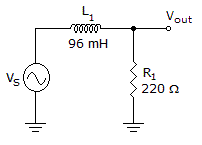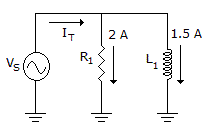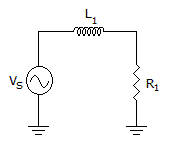# Electronics - RL Circuits

### Exercise :: RL Circuits - Filling the Blanks

6.

The impedance of a series circuit when R = 15 kand XL = 10 kis approximately equal to ________.

 A. 5 kB. 6 kC. 18 kD. 25 kExplanation:

No answer description available for this question. Let us discuss.

7.The given circuit is a _______.

 A. low-pass filter B. high-pass filter C. band-pass filter D. band-stop filter

Explanation:

No answer description available for this question. Let us discuss.

8.If the frequency decreases in the given circuit, then _____.

 A. IT decreases B. IR increases C. IL increases D. none of the currents will change

Explanation:

No answer description available for this question. Let us discuss.

9.The cutoff frequency of the circuit in the given circuit is approximately equal to _____.

 A. 21 Hz B. 60 Hz C. 0 Hz D. 365 Hz

Explanation:

No answer description available for this question. Let us discuss.

10.In the circuit given is, _____.

 A. VR leads VL B. VR lags VL C. VR is in phase with VL D. VR, VL, and VS are all in phase